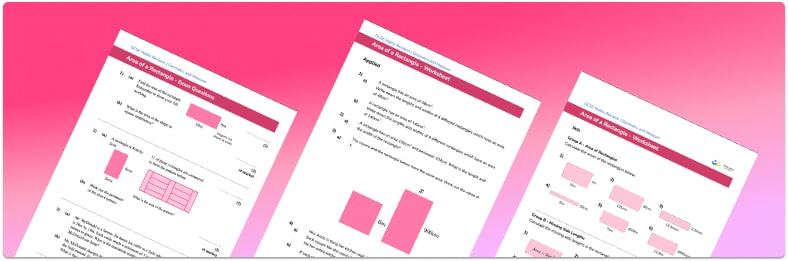# Area Of A Rectangle Worksheet• Section 1 of the printable area of a rectangle worksheet contains 15 skills-based area of a rectangle questions, in 3 groups to support differentiation
• Section 2 contains 4 applied area of a rectangle questions with a mix of worded problems and deeper problem solving questions
• Section 3 contains 4 foundation and higher level GCSE exam style questions
• Answers and a mark scheme for all questions are provided
• Questions follow variation theory with plenty of opportunities for students to work independently at their own level
• All questions created by fully qualified expert secondary maths teachers
• Suitable for GCSE maths revision for AQA, OCR and Edexcel exam boards
• Practice worksheets are also available for related topics such as multiplying fractions and multiplying decimals

• This field is for validation purposes and should be left unchanged.

You can unsubscribe at any time (each email we send will contain an easy way to unsubscribe). To find out more about how we use your data, see our privacy policy.

### Area of a rectangle at a glance

Area is the amount of space covered by a 2D shape and is measured in square units – metric units of area include mm2, cm2 and  m2. In the simplest case, a square or a rectangle may be drawn on a centimetre squared grid. The area of the square or the area of the rectangle can be found by counting squares inside the shape.

To work out the area of a rectangle, we use the formula length width. The area of the square is calculated in the same way – but as the side lengths of a square are all equal, we’re essentially squaring the side length. The formulae for the area of triangles and the area of quadrilaterals such as parallelograms follow from this.

Sometimes students are given the total area of a rectangle or square, and are asked to find a missing side. To do this, divide the area by the given side length. This can require learners to work with decimals so it is important these skills are secure. They may also need to convert between different metric units – for example, if one side is given in centimetres and the other in millimetres.

The area of rectilinear shapes (shapes with straight sides and right angles) can be found by splitting the shape into separate squares or rectangles and finding the area of each one.

In addition to using integers for side lengths, decimals and fractions are also common when calculating area. Students may also be asked to apply multiplication of fractions to the area of a square or rectangle. Students can often confuse finding area with the perimeter of a rectangle with finding the area of a rectangle so it is important to have a strong understanding of these skills.

Looking forward, students can then progress to additional area worksheets and other geometry worksheets, for example an angles in polygons worksheet or area and circumference of a circle worksheet.For more teaching and learning support on Geometry our GCSE maths lessons provide step by step support for all GCSE maths concepts.

## Do you have KS4 students who need more focused attention to succeed at GCSE?There will be students in your class who require individual attention to help them succeed in their maths GCSEs. In a class of 30, it’s not always easy to provide.

Help your students feel confident with exam-style questions and the strategies they’ll need to answer them correctly with our dedicated GCSE maths revision programme.

Lessons are selected to provide support where each student needs it most, and specially-trained GCSE maths tutors adapt the pitch and pace of each lesson. This ensures a personalised revision programme that raises grades and boosts confidence.

Find out more